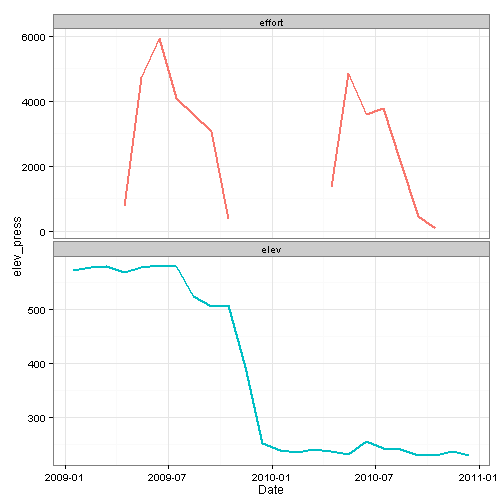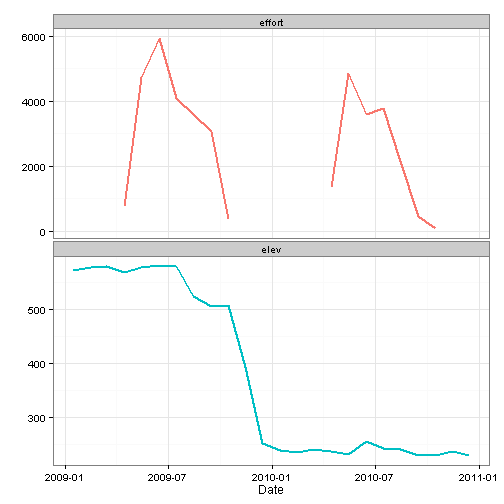# Multiple plots in ggplot2

The RMarkdown source to this file can be found here

As I have mentioned previously is that I use ggplots a ton in my work. It is my goto for plotting in R and I have really loved the ease of plotting with this package. One thing that can see kind of tricky is plotting multiple panels in a single figure. There are a couple of different ways to do this using ggplot2 and the gridExtra packages.

### ggplot2 and facet_wrap

Within ggplot2 there is `facet_wrap` which will allow you to create multiple panels by a variable with a single line of code.

As an example:The `facet_wrap` command allows you to wrap panels by a single variable using the `~x` command, where `x` is your by variable. You can specify the number of columns via `ncol`. One thing thing to notice that the x and y axis are equal among the facets. You can allow these to be vary among the different facets using `scales` command. You can set it to `scales = 'free_x` to allow the x-axis to be variable but constant y, `scales = 'free_y` to allow the y-axis to be variable but constant x, and `scales = 'free` for both to vary.

Now this works pretty well when you have a dataset up already like this, but what if you have data from two different datasets?

Lets load some data used to generate plot of angling effort and the surface area of a reservoir.

For you to be able to use `ggplot` you will need to get the data into a single dataset and as you can tell from above that the column headers do no align (except for date). To get this into single dataset to be used in ggplot takes a couple of steps.

and lets plot the data using `ggplot`As you might be able to tell from the above figure, there is a problem with the above figure as the y-axis label is not appropriate and probably should not be included. The option in this case is to remove it and add it in with another program.Another issue with this figure is that tehre are no ticks in the upper figure of effort. You could do do `scales = 'free'` but this would add in all the dates again along the x-axis. The other option is to use create to seperate ggplot objects and align them with `grid.arrange` in the gridExtra package.

### grid.arrangeNow as you can see in the above figure that this is much closer to what I would normally like in a figure, except that because effort and surface area have a differing number of digits, the figures do not fully align.

You can work around this using the gtable package.The above figure then no longer needs another program to add the axis labels and the top facet has tick marks.

Tags:

Categories:

Updated: